# 2nd exampleThe structural frame
The construction
The reinforcement I
The reinforcement II
Quantity/Cost estimation
Detailing drawings
Introduction >Wind and Seismic Forces >
Structural model and Analysis
Slabs
Seismic behavour of frames
Appendix A
Appendix B
Appendix C
Appendix D
Introduction >
Modelling slabsMaterials
To be continued >
Introduction

## 2nd example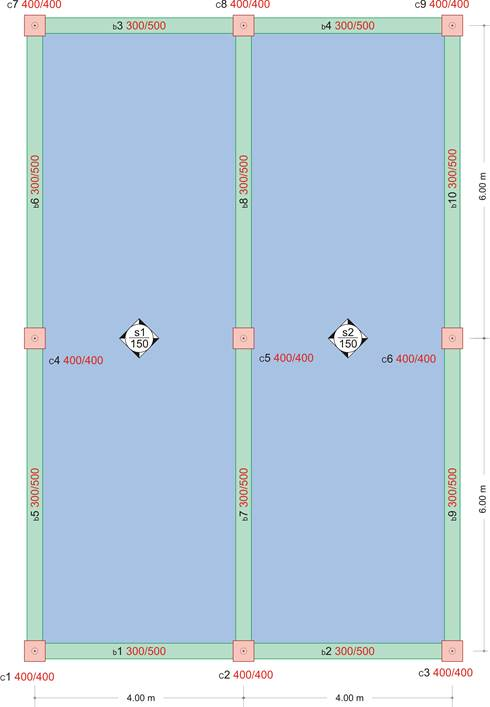project < Β_422-2>

The structure of this example derives from 1st example by removing the two middle beams. The aspect ratio for both slabs is ε=Ly/Lx=12.0/4.0=3.0> 2.0, therefore they can be treated and analysed as two continuous one-way slabs.

## Slab analysis using hand methods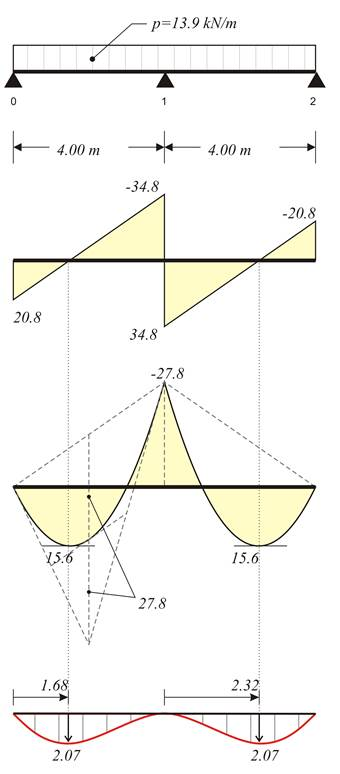For 1.00 m wide slab strip: Self-weight: go=0.15m×1.00m×25.00 kN/m3= 3.75 kN/m, Covering load: ge=1.00 kN/m (given), Total dead load: g=4.75 kN/m, Live load: q=5.00 kN/m (given). Thus, the total load is p=γg×g+γq×q=1.35×4.75+1.50×5.00=13.9 kN/m. The bending moment at the support is calculated using table b3, line 1.   M1=-p×L2/8=-13.9×4.02/8=-27.8 kNm,   V01=p×L/2+M1/L=13.9×4.0/2-27.8/4.0= =20.8 kN V10= p×L/2-M1/L=13.9×4.0/2+27.8/4.0= =34.8 kN   maxM01=V012/(2×p)=20.82/(2×13.9)= =15.6 kNm   According to §4.5.2, from expression (3) → C1=(-13.9×4.03/24+20.8×4.02/6)m2= =18.4 kN×m2 Expression (4) → (13.9/6)z3-(20.8/2)z2-0+18.4=0 → 2.317z3-10.4z2+18.4=0 →zmax=1.68 m (2) → y(z)=1/9.225×[(13.9/24)×1.684-(20.8/6)×1.683+0×1.682+18.4×1,68)] → y(1.68)=2.07 mm

## Slab analysis using software: The actual structure in 3DBefore analysing, set these parameters, as in 1st example: Tab Meshing: “Overall size” = 0.10 m, “Perimeter size” = 0.05 m, Tab Modules: “SLABS” = ON, Tab Loads: “Adverse Slabs” = ΟΝ.

## The deformation of slabs in 3D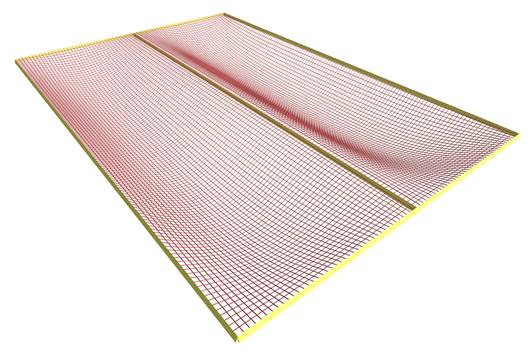For most of the region towards direction y, the slab is curved only along direction x.

## Shear forces from ‘Slab Results’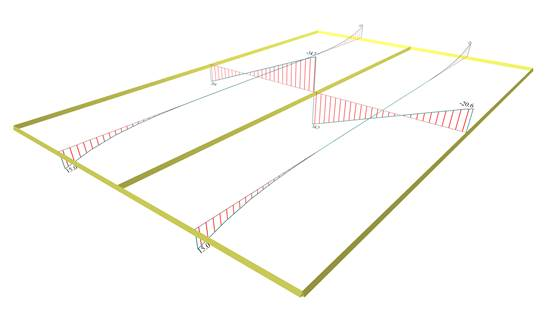to the respective values of the one-way slabs, calculated previously.

Also, shears [Vy] extend in an area near the supports with maximum value of 15.0 kN.

## The 3D distribution of shear forces [Vx]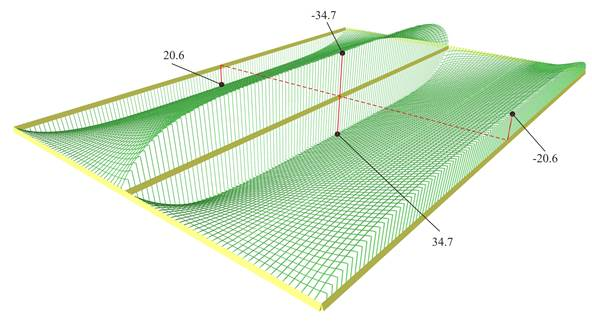In the largest part, it matches the corresponding distribution of one-way slabs.

## The 3D distribution of shear forces [Vy]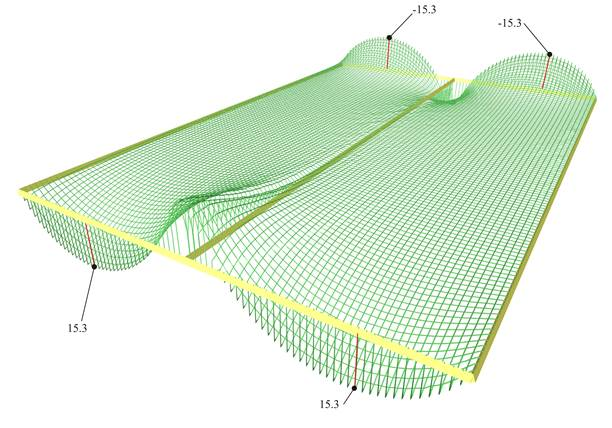Shear forces [Vy] extended only to the regions of end supports.

## The bending moments from ‘Slabs Results’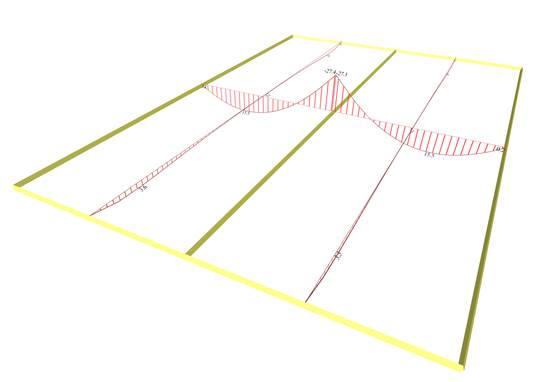Notice that in the middle cross-section, the moments [Mx] 15.5 and -27.4 kNm, are equal to the values of the one-way slabs calculated previously.

On the other hand, moments [My] do exist, 3.6 kNm, but they are insignificant.

## The 3D distribution of bending moments [Mx]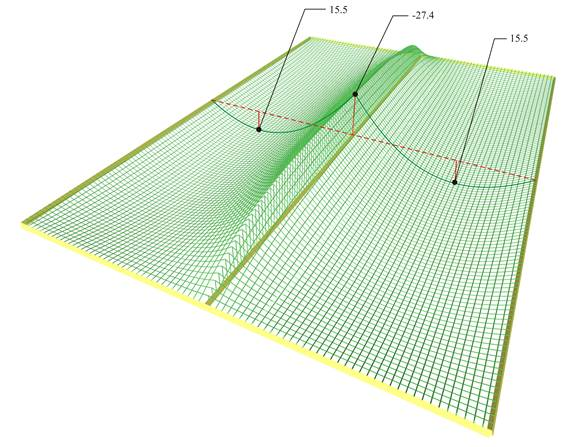Along most of the region, it resembles the corresponding distribution of one-way slabs.

## The 3D distribution of bending moments [My]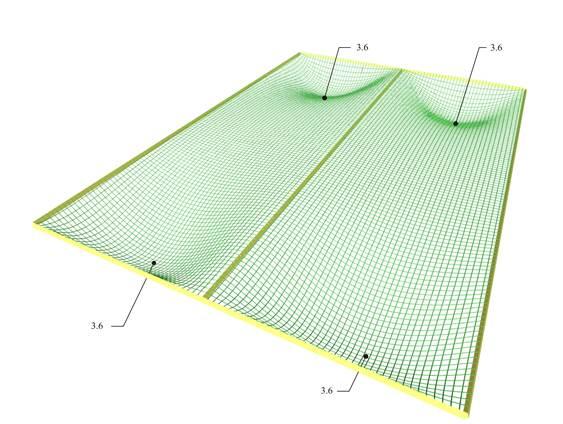Bending moments [My] are small and extended only in the regions of end supports.

## Deflection from ‘Slab Results’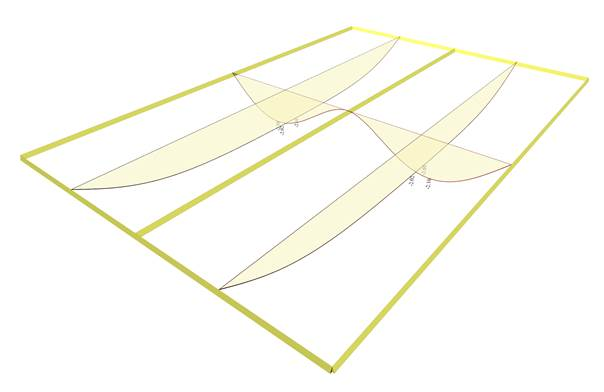Notice that in the middle cross-section, the deflection is 2.10 mm, that is equal to the respective deflection of the one-way slabs calculated previously.